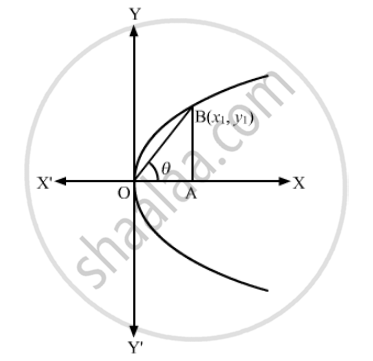Advertisement Remove all ads

# Find the Length of the Line Segment Joining the Vertex of the Parabola Y2 = 4ax and a Point on the Parabola Where the Line-segment Makes an Angle θ to the X-axis. - Mathematics

Find the length of the line segment joining the vertex of the parabola y2 = 4ax and a point on the parabola where the line-segment makes an angle θ to the x-axis.

Advertisement Remove all ads

#### SolutionLet the coordinates of the point on the parabola be B (x1y1).
Let BO be the line segment
In right triangle AOB

$cos\theta = \frac{AO}{OB} \text{ and sin }\theta = \frac{AB}{OB}$
$\Rightarrow cos\theta = \frac{x_1}{OB} \text{ and sin }\theta = \frac{y_1}{OB}$

∴ x1 = OBcosθ and y1 = OBsinθ
Now, the curve is passing through (x1y1)
∴ (y1)2 = 4a(x1)
⇒( OBsinθ)2 = 4a(OBcosθ)

$\Rightarrow {OB}^2 \sin^2 \theta = 4aO\text{ Bcos }\theta$
$\Rightarrow OB = \frac{4acos\theta}{\sin^2 \theta} = 4a\text{ cosec }\theta . \cot\theta$

Is there an error in this question or solution?
Advertisement Remove all ads

#### APPEARS IN

RD Sharma Class 11 Mathematics Textbook
Chapter 25 Parabola
Exercise 25.1 | Q 15 | Page 25
Advertisement Remove all ads
Advertisement Remove all ads
Share
Notifications

View all notifications

Forgot password?